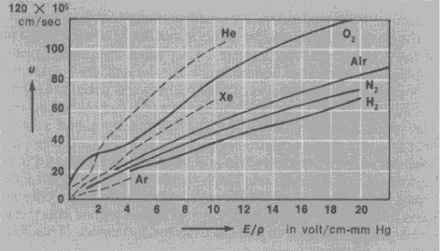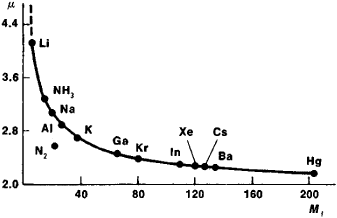# Mobility of Ions and Electrons

## Mobility of Ions and Electrons

(1) The mobility of ions or electrons in a gas or a low-temperature plasma is the ratio of the mean velocity u of the directed motion of the electrons or ions (directed as a result of the action of an electric field) to the electric field strength E: μ = u/E. In principle, the dependence of u on E is given by the solution of the Boltzmann kinetic equation. Not only the solution, however, but even writing down this equation correctly involves considerable difficulties resulting from the diversity of the elementary processes in which ions and electrons are participating. For this reason, the mobility is usually calculated theoretically, on an approximate basis, by introducing simplifying assumptions. Ionic mobility μ,• and electron mobility μe are studied separately, since different elementary processes determine the motion of ions and electrons. It should be noted that electrons, because of their small mass, lose only an insignificant part of their energy in elastic collisions. Even in weak fields their acquisition of directed motion, which is superimposed on thermal, or random, motion, therefore results in their average energy being much greater than the energy of heavy neutral atoms and molecules.

The mobility of ions and electrons was first analyzed theoretically by P. Langevin in 1903. More rigorous and complex theories describing the dependence of u on E were subsequently developed. The first measurement of μe was made by the British physicist J. Townsend when he studied the diffusion of a beam of electrons moving in an electric field and the displacement of this beam in a magnetic field. The most accurate data on the dependence of u on E are presented in Figure 1. Approximate values for μe are obtained by measuring E and the concentration and mobility of the electrons in the positive column of an electrical discharge in a gas.Figure 1. Dependence of u on the ratio E/p for different gases: (u) velocity of the directed motion of electrons under the action of the electric field E, (p) gas pressure referred to the equivalent pressure at 0° C

The mobility of ions moving in a foreign gas is described satisfactorily by Langevin’s theory, according to which the mobility in a given gas depends only on the mass of the ion (Figure 2). The main process determining the μ of the ions in a partially ionized gas is ion charge exchange. After traversing the charge-exchange mean free path, an ion exchanges charge with a neutral particle, and the newly produced ion moves off with an initial velocity close to the thermal velocity—this is the relay-type mechanism of ionic motion. In this case in strong fields u ≈ (E/p)½, where ρ is the gas pressure, referred to the equivalent pressure at 0°C. The development of this theory has permitted the thermal motion of neutral atoms or molecules to be taken into account. In extremely weak fields the theory predicts, and experiment confirms, a linear dependence of the u of ions on E.Figure 2. Dependence of ionic mobility μ on ionic mass M1

The relationship between the mobility of ions or electrons and the diffusion coefficient D is given by the Einstein relation D/μ = kT/e. Here T is the absolute temperature of the charged particles, which are assumed to have a Maxwellian distribution (in a mixture of different charged and neutral particles the average energies and, consequently, the temperatures of the particles may be different—this is the property of the nonisothermicity of such a mixture); k is the Boltzmann constant; and e is the charge of an electron.

(2) The mobility of ions in solutions is U = Fu, where F is the Faraday constant and u is the velocity of an ion in cm/sec for an electric field strength of 1 volt/cm. The quantity U depends on the nature of the ion and on the temperature, dielectric constant, viscosity, and concentration of the solution.

L. A. SENA

Site: Follow: Share:
Open / Close